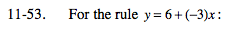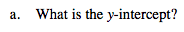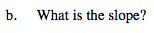### Home > MC2 > Chapter 11 > Lesson 11.2.3 > Problem11-53

11-53.The y-intercept is the point at which the graph intersects the y-axis. The graph will intersect the y-axis when x = 0.

Substitute 0 for x in the expression.
y = 6 + (−3)(0)

y = 6The slope is the value that y increases or decreases when x increases by one.

Create a table of values or sketch the graph on graph paper.

You should notice that from x = 0 to x = 1 the y-value decreases by 3. What is the slope?